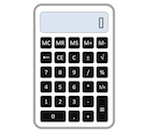### Handy Conversion Formulas for Process ControlHere are very handy conversion calculations for engineering and process control. You may want to bookmark this page for fast lookup.

Area
• 1 acre = 43560 square feet = 4840 square yards = 4046.86 square meters
Distance
• 1 inch = 2.54 centimeters 1 foot = 12 inches
• 1 yard  = 3 feet
• 1 mile  = 5280 feet
Energy
• 1 British thermal unit = 251.996 calories = 1055.06 joules = 1055.06 watt-seconds = 0.293071 watt-hour = 1.05506 x 1010 ergs = 778.169 foot-pound-force
Force
• 1 pound-force = 4.448222 newtons
• 1 kilogram-force = 9.80665 newtons
Mass
• 1 pound-mass = 0.4535924 kilogram
Power
• 1 horsepower = 550 foot-pounds per second = 745.7 watts = 2544.43 British thermal units per hour  = 0.0760181 boiler horsepower
Pressure (Absolute only)
• 1 standard atmosphere = 14.7 pounds per square inch absolute = 101.325 kilopascal absolute = 1.01325 bar absolute = 760 millimeters of mercury absolute = 760 torr
Pressure (all gauge or all absolute)
• 1 pounds per square inch = 2.03602 inches of mercury at 0 deg. C = 27.6799 inches of water at 4 deg. C = 6.894757 kilopascal = 0.06894757 bar
• 1 bar = 100 kilopascal = 14.504 pounds per square inch
• 1 meter of water at 4 deg. C = 9.80665 kilopascal
Temperature
• Fahrenheit = (deg. C)(9/5) + 32
• Celsius = (deg. F − 32)(5/9)
• Rankine = deg. F + 459.67
• Kelvin = deg. C + 273.15
Velocity
• 1 mile per hour= 88 feet per minute= 1.46667 feet per second= 1.60934 kilometer per hour = 0.44704 meter per second = 0.868976 knot
Volume
• 1 gallon = 231.0 cubic inches = 4 quarts = 8 pints = 16 cups = 128 fluid ounces = 3.7854 liters
• 1 milliliter = 1 cubic centimeter